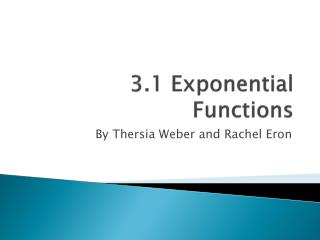# 3.1 Exponential Functions - PowerPoint PPT PresentationDownload Presentation3.1 Exponential Functions

3.1 Exponential FunctionsDownload Presentation## 3.1 Exponential Functions

- - - - - - - - - - - - - - - - - - - - - - - - - - - E N D - - - - - - - - - - - - - - - - - - - - - - - - - - -
##### Presentation Transcript

1. 3.1 Exponential Functions By Thersia Weber and Rachel Eron

2. Introduction

3. Exponential Format Only an exponential function if the x is in the exponent. f(x) = abx a = initial amount b = rate of growth/base x = variable b > 1: exponential growth b < 1: exponential decay

4. Identifying Exponential Functions f(x) = 4x7 Exponential Function? Initial Value? Base? Growth or Decay? f(x) = 3x Exponential Function? Initial Value? Base? Growth or Decay? Yes X is in exponent 1 A isn’t listed, automatically 1 3 B=3 Growth B=3 > 1 No X isn’t in exponent - - -

5. Table of Values a = x = 0 then plug it into y=abx y=6bx then plug in another value on the table 3/2 = 6b1 solve for b b= 3 . 1 2 6 b= 3 = 1 12 4 plug b into equation y= 6(1/4)x Power x f(x) -2 96 -1 24 0 6 1 3/2 2 3/8

6. Table of Values Examples x f(x) -2 108 -1 36 0 12 1 4 2 4/3 x f(x) y = abx y = 4.8bx 5.6 = 4.8b10 1.167 = b10 ln 1.167 = 10 ln b ln b = .0154 b = e.0154 y = 4.8 e.0154x y = abx y = 12bx 4 = 12b1 4/12 = b 4/12 = 1/3 = b y = 12 (1/3)x y = 12 (1/3)x y = 4.8 e.0154x

7. Review of Graph Transformations Graph transformations previously learned also apply to exponential functions http://www.explorelearning.com/index.cfm?method=cResource.dspView&ResourceID=104# Horizontal Translations y = abx-c graph shifts to right y = abx+c graph shifts to left Vertical Translations y = abx +c graph shifts up y = abx-c graph shifts down Axis Flips y = ab-x graph flips over y axis y = -abx graph flips over x axis Use URL to explore transformations of Exponential functions on graph

8. Plugging into Equations Exponential equations can be found in many real life situations. To solve real life equations, look at it as y = abx and find initial amount and other variables. Plug in the numbers given and find the information asked for in problem. The number B of bacteria in a petri dish culture of t hours is given by: B = 100e0.693t Find initial number of bacteria present. How many bacteria are present after 6 hours? B = 100e0.693t B = 100e0.693x6 B = 6394 Initial: 100 # Present after 6 hours: 6394

9. Allowance • Suppose your parents are going to pay you a weekly allowance for doing your household chores this coming year. Also, imagine that they are going to give you a choice of two payment schemes. With Choice A, your parents will pay you \$10 every week. Therefore, you will have earned \$10 after the first week, \$20 after the second week, \$30 after the third week, and so on. With Choice B, just to be nice, they are going to pay you \$0.01 before you even start working. After you complete chores for week one, they will double your money, thus giving you a total of \$0.02. After you complete chores for week two, they will once again double your money, thus giving you a total of \$0.04. They will continue to double your money at the end of every week. • Write an equation for each payment scheme and use www.graphsketch.com to make graphs. Explain which payment scheme you will choose and why using the graphs. • Each project will be turned in and graded according to rubric, worth 20 points.

10. Allowance Answers • Each payment scheme will give you a fair amount of allowance, but judging by the graphs, the second payment plan would be a better choice because you will earn more eventually, faster. 2-3 groups to present explanation. If present, 5 extra credit points.

11. References • Precalculus Textbook by Franklin Demana, Bert Waits, Bregory Foley, and Daniel Kennedy • www.graphsketch.com (Graph, slide 10) • http://www.explorelearning.com/index.cfm?method=cResource.dspView&ResourceID=104# (Graphing Activity, slide 7) • http://www.cartoonstock.com/directory/e/exponential_growth.asp (Introduction Comic) • http://education.ti.com/calculators/downloads/US/Activities/Detail?id=7727 (Supplemental Activity, Allowance)

12. Quiz • Now take a 10 question assessment on what you’ve learned. • Assessment • Assessment Answers